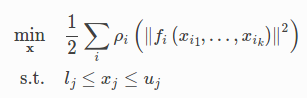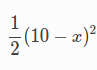# Ceres 函式庫簡介

## 前言

Ceres 是用來求解 Optimization 問題的 C++ 開源函式庫，是由 Google 所釋出的，文件相當完整，而且這個函式庫還有不少 好處(例如 code 品質、使用的方便性等等)。

## 安裝 Ceres (in Linux)

sudo apt-get install liblapack-dev libsuitesparse-dev libcxsparse3.1.2 libgflags-dev libgoogle-glog-dev libgtest-dev


git clone https://github.com/ceres-solver/ceres-solver.git
mkdir ceres-bin
cd ceres-bin
cmake ../ceres-solver
make -j3
make test
sudo make install


## 使用 Ceres 來求解一個超簡單的方程式struct CostFunctor {
template <typename T>
bool operator()(const T* const x, T* residual) const {
residual = T(10.0) - x;
return true;
}
};


#include "ceres/ceres.h"
#include "glog/logging.h"

//會使用到的 function
using ceres::AutoDiffCostFunction;
using ceres::CostFunction;
using ceres::Problem;
using ceres::Solver;
using ceres::Solve;

// 第一步要先定義的 functor
struct CostFunctor {
template <typename T> bool operator()(const T* const x, T* residual) const {
residual = 10.0 - x;
return true;
}
};

int main(int argc, char** argv) {

// 設定想要求解的變數初始值
double x = 0.5;
const double initial_x = x;

// 初始化 problem
Problem problem;

// 定義 cost_function，這邊是用到 AutoDiffCostFunction
// AutoDiffCostFunction 會吃上面定義的 functor，然後用 functor 裡寫的方式算誤差
CostFunction* cost_function =
new AutoDiffCostFunction<CostFunctor, 1, 1>(new CostFunctor);
// 定義 problem 要使用我們剛建立的 cost_function

// Run solver 來解 problem
Solver::Options options;
options.minimizer_progress_to_stdout = true;
Solver::Summary summary;
Solve(options, &problem, &summary);

// 輸出結果
std::cout << summary.BriefReport() << "\n";
std::cout << "x : " << initial_x
<< " -> " << x << "\n";

return 0;
}


1. 定義 functor
2. 定義欲求參數的初始值
3. 定義 cost_function 並指定 problem 要使用 cost_function 求解
4. 設定並執行 Solver 求解問題
5. 看結果

iter      cost      cost_change  |gradient|   |step|    tr_ratio  tr_radius  ls_iter  iter_time  total_time
0  4.512500e+01    0.00e+00    9.50e+00   0.00e+00   0.00e+00  1.00e+04        0    7.54e-05    1.94e-03
1  4.511598e-07    4.51e+01    9.50e-04   9.50e+00   1.00e+00  3.00e+04        1    8.24e-04    3.01e-03
2  5.012552e-16    4.51e-07    3.17e-08   9.50e-04   1.00e+00  9.00e+04        1    5.95e-05    3.16e-03
Ceres Solver Report: Iterations: 3, Initial cost: 4.512500e+01, Final cost: 5.012552e-16, Termination: CONVERGENCE
x : 0.5 -> 10


## 延伸閱讀

@pojenlai 演算法工程師，對機器人跟電腦視覺有少許研究，最近在學習看清事物的本質與改進自己的觀念